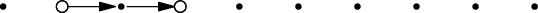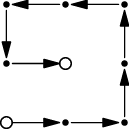# Internet Problem Solving Contest

## Problem G – Grid travel

You are given a rectangular grid consisting of r × c points. The lower left corner has coordinates (1,1), the upper right corner has coordinates (c,r). The neighbors of a point (x,y) are the points (x 1,y), (x + 1,y), (x,y 1), and (x,y + 1), if they exist. A path is a sequence of points such that subsequent points are neighbors and each point appears on the path at most once.

Problem speciﬁcation

Given two distinct points (xs,ys) and (xf,yf), ﬁnd one longest path from (xs,ys) to (xf,yf).

Input speciﬁcation

The ﬁrst line of the input ﬁle contains an integer t specifying the number of test cases. Each test case is preceded by a blank line.

Each test case consists of a single line with six integers – r, c, xs, ys, xf, yf.

For both the easy subproblem G1 and the hard subproblem G2, you may assume that 1 r,c 100 and rc 2. Additionally, for the easy subproblem G1 you may assume that r 5.

Output speciﬁcation

For each test case, output a single string describing one possible longest path. If there are multiple longest paths, output any one of them.

A path a1,a2,,ak is described by a string consisting of k 1 letters U, D, L, R. The i-th letter in the string describes the move from point ai to ai+1:
If ai = (x,y) and ai+1 = (x,y + 1), the i-th letter should be U.
If ai = (x,y) and ai+1 = (x,y 1), the i-th letter should be D.
If ai = (x,y) and ai+1 = (x + 1,y), the i-th letter should be R.
If ai = (x,y) and ai+1 = (x 1,y), the i-th letter should be L.

Example

input
2

1 10 2 1 4 1

3 3 1 1 2 2
output
RR
RRUULLDR

ﬁrst example:second example: